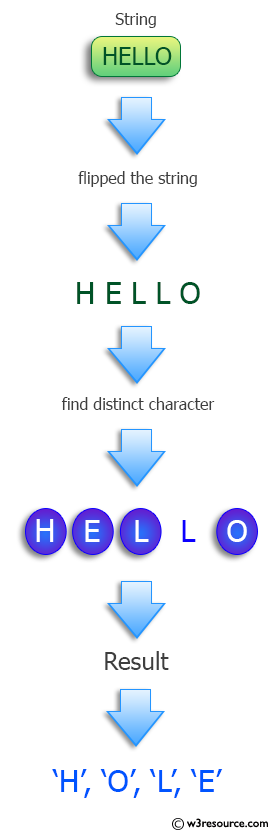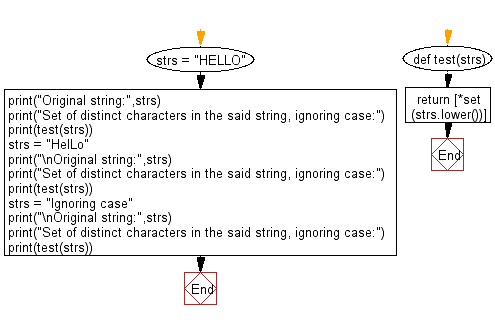﻿ Python: Find the set of distinct characters in a string, ignoring case - w3resource# Python: Find the set of distinct characters in a string, ignoring case

## Python Programming Puzzles: Exercise-42 with Solution

Write a Python program to find the set of distinct characters in a given string, ignoring case.

```Input: HELLO
Output:
['h', 'o', 'l', 'e']

Input: HelLo
Output:
['h', 'o', 'l', 'e']

Input: Ignoring case
Output:
['s', 'n', 'c', 'o', 'e', 'i', 'r', 'g', 'a', ' ']
```

Pictorial Presentation:Sample Solution-1:

Python Code:

``````#License: https://bit.ly/3oLErEI

def test(strs):
return [*set(strs.lower())]

strs = "HELLO"
print("Original string:",strs)
print("Set of distinct characters in the said string, ignoring case:")
print(test(strs))
strs = "HelLo"
print("\nOriginal string:",strs)
print("Set of distinct characters in the said string, ignoring case:")
print(test(strs))
strs = "Ignoring case"
print("\nOriginal string:",strs)
print("Set of distinct characters in the said string, ignoring case:")
print(test(strs))
``````

Sample Output:

```Original string: HELLO
Set of distinct characters in the said string, ignoring case:
['o', 'e', 'l', 'h']

Original string: HelLo
Set of distinct characters in the said string, ignoring case:
['o', 'e', 'l', 'h']

Original string: Ignoring case
Set of distinct characters in the said string, ignoring case:
['o', ' ', 'i', 'r', 'e', 'g', 'a', 'n', 'c', 's']
```

Flowchart:## Visualize Python code execution:

The following tool visualize what the computer is doing step-by-step as it executes the said program:

Sample Solution-2:

Python Code:

``````#License: https://bit.ly/3oLErEI

def test(strs):
return list(set(strs.lower()))
strs = "HELLO"
print("Original string:",strs)
print("Set of distinct characters in the said string, ignoring case:")
print(test(strs))
strs = "HelLo"
print("\nOriginal string:",strs)
print("Set of distinct characters in the said string, ignoring case:")
print(test(strs))
strs = "Ignoring case"
print("\nOriginal string:",strs)
print("Set of distinct characters in the said string, ignoring case:")
print(test(strs))
``````

Sample Output:

```Original string: HELLO
Set of distinct characters in the said string, ignoring case:
['h', 'o', 'l', 'e']

Original string: HelLo
Set of distinct characters in the said string, ignoring case:
['h', 'o', 'l', 'e']

Original string: Ignoring case
Set of distinct characters in the said string, ignoring case:
['s', 'n', 'c', 'o', 'e', 'i', 'r', 'g', 'a', ' ']
```

Flowchart:## Visualize Python code execution:

The following tool visualize what the computer is doing step-by-step as it executes the said program:

Python Code Editor :

Have another way to solve this solution? Contribute your code (and comments) through Disqus.

What is the difficulty level of this exercise?

Test your Programming skills with w3resource's quiz.

﻿

## Python: Tips of the Day

Clamps num within the inclusive range specified by the boundary values x and y:

Example:

```def tips_clamp_num(num,x,y):
return max(min(num, max(x, y)), min(x, y))
print(tips_clamp_num(2, 4, 6))
print(tips_clamp_num(1, -1, -6))
```

Output:

```4
-1
```